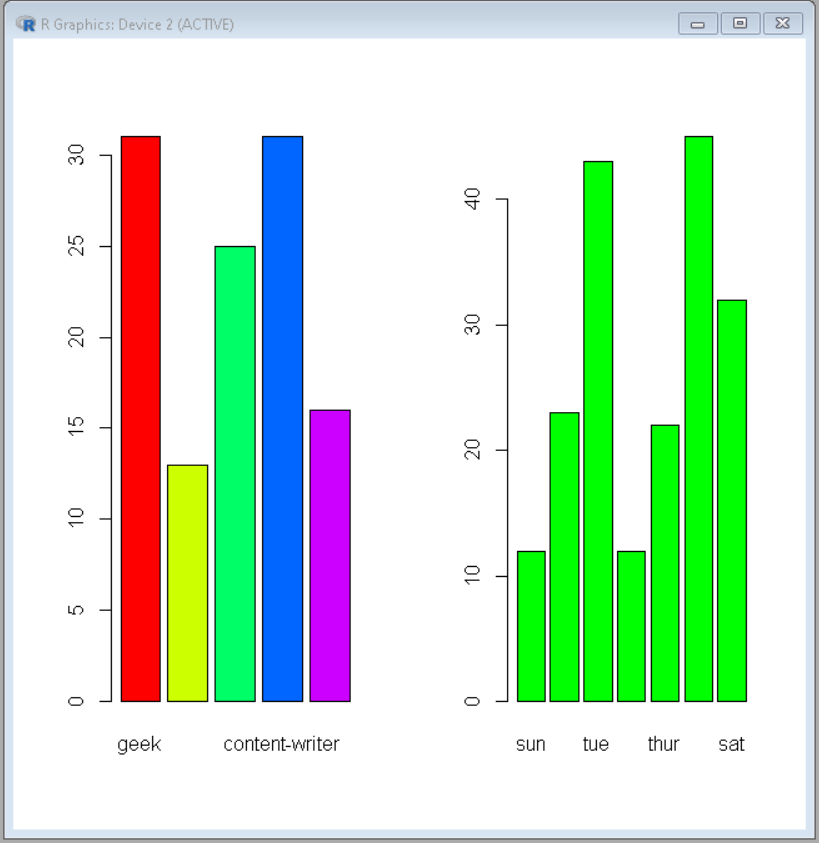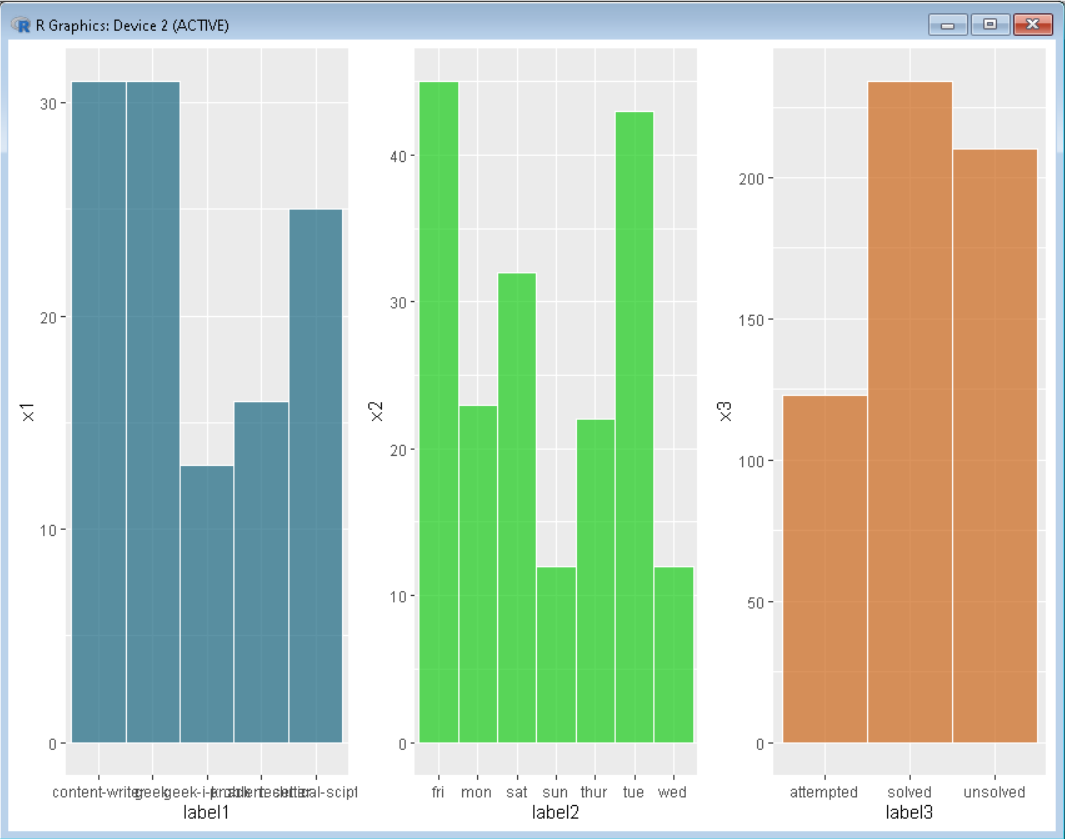Related Articles

# Side by Side bar charts in R

• Last Updated : 21 Jul, 2021

In this article, we will discuss how to draw Bar Charts side by side in R Programming Language.

## Method 1: Using par() function

To draw plots side by side par() function is used.

Syntax:

par(mfrow, mar, mgp, las)

Parameters:

• mfrow- A numeric vector of length 2, which sets the rows and column in which frame has to be divided.
• mar – A numeric vector of length 4, which sets the margin sizes in the following order: bottom, left, top, and right.
• mgp – A numeric vector of length 3, which sets the axis label locations relative to the edge of the inner plot window.
• las – A numeric value indicating the orientation of the tick mark labels and any other text added to a plot after its initialization.

The plots are drawn normally and independent of others. For drawing them side-by-side pass the number of rows and columns as if a grid is being defined.

Example: Plotting bar plots side by side using basic R

## R

 `# Define data-set columns``x1 <- ``c``(31,13,25,31,16)``x2 <- ``c``(12,23,43,12,22,45,32) `` ` `label1 <- ``c``(``'geek'``,``'geek-i-knack'``,``'technical-scipter'``,``            ``'content-writer'``,``'problem-setter'``)``label2 <- ``c``(``'sun'``,``'mon'``,``'tue'``,``'wed'``,``'thur'``,``'fri'``,``'sat'``)  `` ` `# set the plotting area into a 1*2 array``par``(mfrow=``c``(1,2))    `` ` `# Draw the two bar chart using above datasets``barplot``(x1, names.arg = label1,col=``rainbow``(``length``(x1)))``barplot``(x2, names.arg = label2,col =``"green"``)`

Output:## Method 2: Using ggplot2

In this grid.arrange() is used to arrange the plots on a frame.

Syntax:

grid.arrange(plot, nrow, ncol)

Parameter:

• plot- ggplot2 plot which we want to arrange
• nrow- Number of rows
• ncol- Number of columns

Here, plots are drawn normally and independently. Then, the function is called with these plots and the number of rows and columns in a way that a grid is being defined.

Example: Plotting multiple plots side by side using ggplot.

## R

 `# Define data-set columns``x1 <- ``c``(31,13,25,31,16)``x2 <- ``c``(12,23,43,12,22,45,32) ``x3 <- ``c``(234,123,210)`` ` `label1 <- ``c``(``'geek'``,``'geek-i-knack'``,``'technical-scipter'``,``            ``'content-writer'``,``'problem-setter'``)``label2 <- ``c``(``'sun'``,``'mon'``,``'tue'``,``'wed'``,``'thur'``,``'fri'``,``'sat'``)  ``label3 <- ``c``(``'solved'``,``'attempted'``,``'unsolved'``)`` ` `# Create data frame using above ``# data column``data1 <- ``data.frame``(x1,label1)``data2 <- ``data.frame``(x2,label2)``data3 <- ``data.frame``(x3,label3)`` ` `# set the plotting area into a 1*3 array``par``(mfrow=``c``(1,3))    `` ` `# import library ggplot2 and gridExtra``library``(ggplot2)``library``(gridExtra)`` ` `# Draw the three bar chart using above datasets``plot1<-``ggplot``(data1, ``aes``(x=label1, y=x1)) + ``geom_bar``(stat=``"identity"``, width=1, color=``"white"``,``         ``fill=``rgb``(0.1,0.4,0.5,0.7))`` ` `plot2<-``ggplot``(data2, ``aes``(x=label2, y=x2)) + ``geom_bar``(stat=``"identity"``, width=1, color=``"white"``,``         ``fill=``rgb``(0.1,0.8,0.1,0.7))`` ` `plot3<-``ggplot``(data3, ``aes``(x=label3, y=x3)) +``geom_bar``(stat=``"identity"``, width=1, color=``"white"``, ``         ``fill=``rgb``(0.8,0.4,0.1,0.7))`` ` `# Use grid.arrange to put plots in columns``grid.arrange``(plot1, plot2, plot3, ncol=3)`

Output:My Personal Notes arrow_drop_up